# UNIT 6: Gaseous State - Online Test

Q1. Use of hot air balloon in sports at meteorological observation is an application of
Explaination / Solution:
No Explaination.

Q2.

The table indicates the value of van der Waals constant ‘a’ in (dm3)2atm. mol-2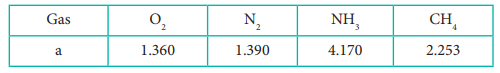The gas which can be most easily liquefied is
Explaination / Solution:

Higher the value of 'a', greater the intermolecular force of attraction, easier the liquefaction.

Q3. Consider the following statements

i. Atmospheric pressure is less at the top of a mountain than at sea level

ii. Gases are much more compressible than solids or liquids

iii. When the atmospheric pressure increases the height of the mercury column rises Select the correct statement

Explaination / Solution:
No Explaination.

Q4. Compressibility factor for CO2 at 400 K and 71.0 bar is 0.8697. The molar volume of CO2 under these conditions is
Explaination / Solution: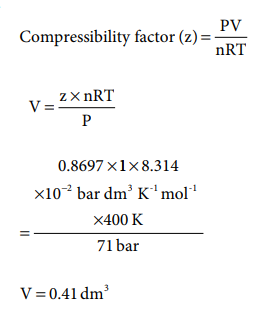Q5. If temperature and volume of an ideal gas is increased to twice its values, the initial pressure P becomes
Explaination / Solution: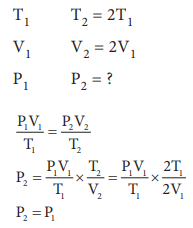Q6. At identical temperature and pressure, the rate of diffusion of hydrogen gas is 3√3 times that of a hydrocarbon having molecular formula CnH2n–2. What is the value of n ?
Explaination / Solution: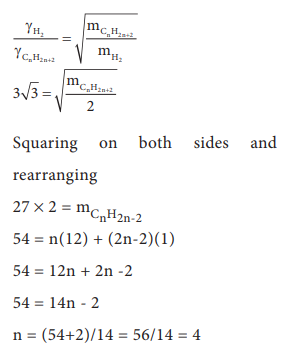Q7. Equal moles of hydrogen and oxygen gases are placed in a container, with a pin-hole through which both can escape what fraction of oxygen escapes in the time required for one-half of the hydrogen to escape.
Explaination / Solution: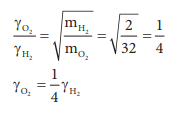The fraction of oxygen that escapes in the time required for one half of the hydrogen to escape is 1/8

Q8. The variation of volume V, with temperature T, keeping pressure constant is called the coefficient of thermal expansion ie α = 1/V (∂V/∂T)P. For an ideal gas α is equal to
Explaination / Solution: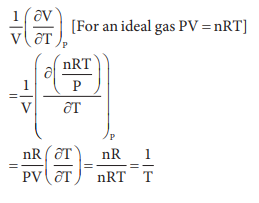Q9. Four gases P, Q, R and S have almost same values of 'b' but their 'a' values (a, b are Vander Waals Constants) are in the order Q < R < S < P. At a particular temperature, among the four gases the most easily liquefiable one is
Explaination / Solution:

Greater the 'a' value, easier the liquefaction

Q10. Maximum deviation from ideal gas is expected from
Explaination / Solution:
No Explaination.